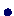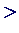# Making decisionsIf we change the test program to create a different quadratic equation:

we get the following output:

2.0*x^2 + 10.0*x + -12.0

Not quite, what we wanted!First we want to input different equations without recompilation. This leads to a new version of TestQuadraticEquation: TestQuadraticEquation.javaAn array is a data structure that combines several elements of the same base type. An array of NUMBER elements is created with

BASETYPE[] myArray;
myArray = new BASETYPE[NUMBER];

• The elements can be accessed with

myArray, myArray ... myArray[NUMBER-1]We create an array of 3 integers and set its values:

integer[] vec = new integer;
vec = 42;
vec = 28;
vec = vec + vec;   //  = 70A Java program can be called with additional parameters, separated by spaces, like

java MYCLASS PAR1 PAR2 .. PARN

The parameters are collected in an array of Strings and passed as argument to the main method.If we start our example with

the argument array arg has the values

arg = "2.0"
arg = "-10.0"
arg = "3"The static method parseDouble of class Double does its best to translate a String into a double value.If TestQuadraticEquation is called with less than 3 parameters or if one of the parameters can't be transformed to a number, we get an error message.Now we extend QuadraticEquation to be able to print negative coefficients: QuadraticEquation.javaThe translation of a double value to a String works different for positive and negative numbers: negative doubles start with a minus sign, positive don't start with a plus sign.

The method toSignedString returns a double with plus or minus sign and extra space. It works by using always a positive value for the translation and adding the sign explicitly.

To do this, we need a way to decide between the values of the double.Decisions can be programmed with the alternative, written as

if (CONDITION) {
DO SOMETHING
} else {
DO SOMETHING ELSE
}

• CONDITION is a logical (boolean) expression, i.e. it can only be true or false.Special boolean expressions are made up from comparisons:

 expression meaning (d0) greater than (d= 0) less than or equal (d == 0) equal (d != 0) not equalThe method toSignedString is private, which means that it can be used only for internal purposes of the class (for the print method in our example).Peter Junglas 8.3.2000